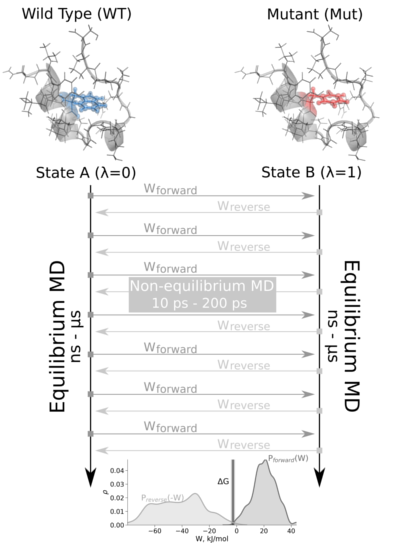# Mutation Free Energy Calculations using BioExcel Building Blocks (biobb)¶

Based on the official pmx tutorial.

This tutorial aims to illustrate how to compute a fast-growth mutation free energy calculation, step by step, using the BioExcel Building Blocks library (biobb). The particular example used is the Staphylococcal nuclease protein (PDB code 1STN), a small, minimal protein, appropriate for a short tutorial.

The non-equilibrium free energy calculation protocol performs a fast alchemical transition in the direction WT->Mut and back Mut->WT. The two equilibrium trajectories needed for the tutorial, one for Wild Type (WT) and another for the Mutated (Mut) protein (Isoleucine 10 to Alanine -I10A-), have already been generated and are included in this example. We will name WT as stateA and Mut as stateB.The tutorial calculates the free energy difference in the folded state of a protein. Starting from two 1ns-length independent equilibrium simulations (WT and mutant), snapshots are selected to start fast (50ps) transitions driving the system in the forward (WT to mutant) and reverse (mutant to WT) directions, and the work values required to perform these transitions are collected. With these values, Crooks Gaussian Intersection (CGI), Bennett Acceptance Ratio (BAR) and Jarzynski estimator methods are used to calculate the free energy difference between the two states.

Please note that for the sake of disk space this tutorial is using 1ns-length equilibrium trajectories, whereas in the original example the equilibrium trajectories used were obtained from 10ns-length simulations.

## Settings¶

### Biobb modules used¶

• biobb_pmx: Tools to setup and run Alchemical Free Energy calculations.
• biobb_gromacs: Tools to setup and run Molecular Dynamics simulations.
• biobb_analysis: Tools to analyse Molecular Dynamics trajectories.

### Auxiliar libraries used¶

• nb_conda_kernels: Enables a Jupyter Notebook or JupyterLab application in one conda environment to access kernels for Python, R, and other languages found in other environments.
• jupyter_contrib_nbextensions: Contains a collection of community-contributed unofficial extensions that add functionality to the Jupyter notebook.
• ipywidgets: Interactive HTML widgets for Jupyter notebooks and the IPython kernel.
• os: Python miscellaneous operating system interfaces
• plotly: Python interactive graphing library integrated in Jupyter notebooks.

### Conda Installation and Launch¶

```git clone https://github.com/bioexcel/biobb_wf_pmx_tutorial.git
cd biobb_wf_pmx_tutorial
conda env create -f conda_env/environment.yml
conda activate biobb_wf_pmx_tutorial
jupyter nbextension enable python-markdown/main
jupyter-notebook biobb_wf_pmx_tutorial/notebooks/biobb_wf_pmx_tutorial.ipynb
```

Please execute the following command before launching the Jupyter Notebook if you experience some issues with widgets:

```jupyter-nbextension enable --py --user widgetsnbextension
```